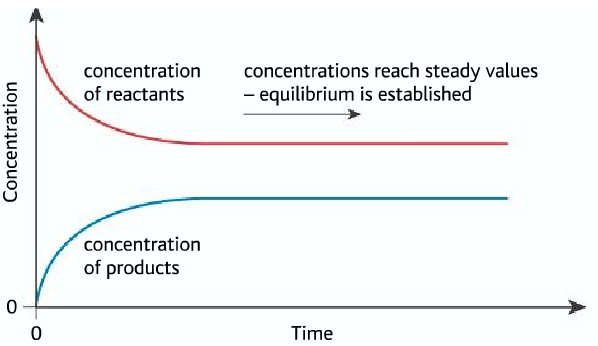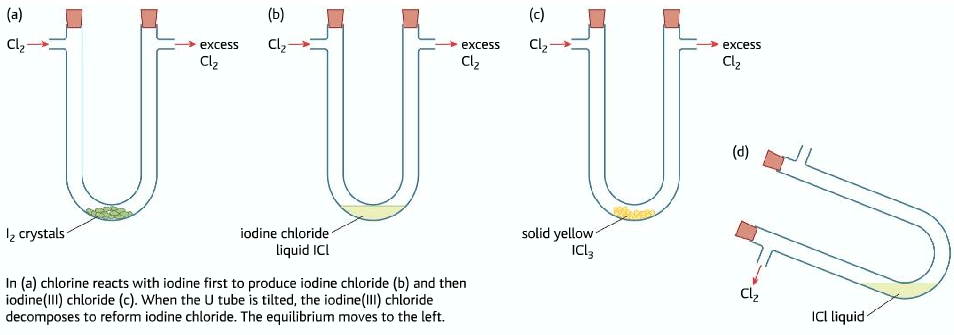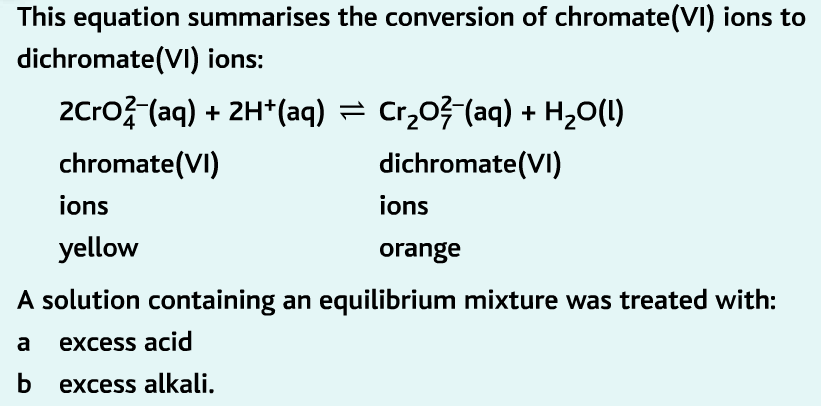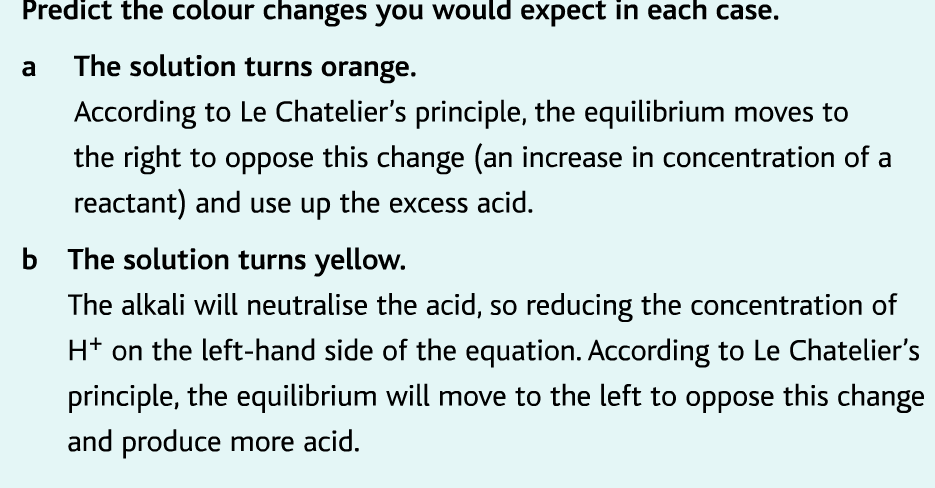# The Chemical Equilibrium or Dynamic Equilibrium

The equilibrium is a state which some reactions reach

It is also called dynamic equilibrium because both forward and backward reactions are going on simultaneously

A+B ⇌ C+D

## Features of dynamic equilibrium

• The rate of both forward and backward reaction are equal
• The amount of reactants and products are constant

## How the dynamic equilibrium is established• At first, the rate of forward reaction is the highest and the rate of the backward reaction is zero.
• As the reaction progresses the rate of forward reaction decreases and the rate of the backward reaction increases.
• The equilibrium is reached when the rate of both forward and backward reaction becomes equal.• At the beginning, the reactants are turning to products so the concentration of reactants are decreasing.
• The reactants are producing products so the concentration of product is increasing.
• When the equilibrium is reached, the rate of forward and backward reaction becomes equal. So the concentration of reactants and products becomes constant.

## Factors which affects the equilibrium position

### Le Chatelier’s Principle

Whenever a system which is in dynamic equilibrium is disturbed it tend to respond in such a way as to oppose the disturbance and so restore equilibrium.

## Factors affecting the equilibrium position

• Temperature
• Pressure
• Concentration

### Temperature

Suppose:

A+B⇌ C+D ΔH= -90KJmol-1

• The enthalpy change given is for the forward reaction
• Therefore the enthalpy change for the backward reaction is +90 KJmol-1
• If the temperature is increased the equilibrium would want to decrease the temperature by absorbing heat, by doing the endothermic reaction. Hence the equilibrium position would shift to the left.
• If the temperature is decreased, the equilibrium would want to increase the temperature, by doing the exothermic reaction. Hence shifting the equilibrium to the right.

### Pressure

• It is a factor for the equilibrium position if anyone of the products or reactants are gas.

Example: A(g) + 2B(g) → C(g) + D(g)

• An increase in pressure would shift the equilibrium position to the right because there are more number of gaseous molecules on the right-hand side ( 3 molecules)

### Concentration

A+B ⇌ C+ D

• If the concentration of B is increased, the equilibrium would shift to the right in order to decrease the concentration of B
• If the concentration of C is increased, the equilibrium position would shift to the left, to decrease the concentration of C

Examples:

1. 2NO2(g) ⇌ N2O4(g)
• NO2 is brown in color, whereas N2O4 is colorless.
• The equilibrium mixture is light brown in color, as there are both gases present.
• If the pressure is increased, the equilibrium shifts to the right, less number of gaseous molecules on right. Producing more colorless N2O4 , turning the mixture lighter in color.
• If the temperature in increased, the equilibrium shifts to the left-hand side, because the forward reaction is exothermic. Producing more brown NO2 , turning the mixture darker.

### Iodine(I)Chloride and Iodine(II)Chloride

• Chloride reacts with iodine to form Iodine(I)Chloride

I2 (s) + Cl2(g) → 2ICl(l)

• If excess chlorine is passed ICl reacts with more chlorine to form Iodine(III)Chloride ICl3

ICl(l) +Cl2(g) ⇌ ICl3(s)

• ICl3 →Yellow crystal

ICl à Brown liquid

• When excess chlorine is passed, the equilibrium shifts to the right producing and more yellow solid is formed.
• When the U-tube is tipped, chlorine gas is removed, the equilibrium shifts to the left, forming more brown liquid.iii) Color Change:1. iv) Methane Hydrate (s) ⇌ methane (g) + water (l)
• The forward reaction is exothermic
• Due to the recent climate change and increase in temperature, the equilibrium is shifting to the right and more methane gas is formed.

## Haber process

• It is the name given for the production of ammonia gas.

N2 + 3H2 ⇌ 2NH3 ΔH = -92 KJmol-1

### Conditions for Haber process

Temperature: 450°C

Pressure: 200 atm

Catalyst used: Iron

#### Reasons for such decision:

Temperature: Even though a low temperature is preferred, but still a high temperature of 450°C is used. Because a low temperature would decrease the rate of reaction. But a temperature higher than 450°C would result in a lower yield which would not be beneficial.

Pressure: A high pressure of 200 atm is understandable as it shifts the equilibrium position to the right. As there are less number of gaseous molecules on the right-hand side. But a higher pressure than 200 atm would be expensive also risky.

Catalyst: The ruthenium is 40% more efficient than iron but still iron is used because ruthenium does not work at a lower temperature.

#### Things done during the Haber process:

• The ammonia gas produced is liquefied and removed. Shifting the equilibrium position to the right producing more ammonia gas.
• The nitrogen and hydrogen gas are recycled continuously, leading to all reactants being used up. Atom economy is therefore 100%.